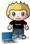# List of links to all the SQL 2008 Spatial Methods

posted in: Uncategorized | 0I always struggle to find the full list of SQL 2008 Spatial methods for Geography when I need them so this is really just a helper post so I can find them again later but maybe it will help you also. Since they are case sensitive and don’t have intelisence I can never remember the syntax.

### Functions

These static methods, implemented in the class Functions, can both be registered in SQL Server and used through T-SQL, as well as be used directly from the CLR:
bool IsValidGeographyFromGeometry(SqlGeometry geometry)
Check if an input geometry can represent a valid geography without throwing an exception.
This function requires that the geometry be in longitude/latitude coordinates and that
those coordinates are in correct order in the geometry instance (i.e. latitude/longitude
not longitude/latitude). This function will return false (0) if the input geometry is not
in the correct latitude/longitude format, including a valid geography SRID.
bool IsValidGeographyFromText(string inputWKT, int srid)
Check if an input WKT can represent a valid geography. This function requires that
the WTK coordinate values are longitude/latitude values, in that order and that a valid
geography SRID value is supplied. This function will not throw an exception even in
edge conditions (i.e. longitude/latitude coordinates are reversed to latitude/longitude).
SqlGeography MakeValidGeographyFromGeometry(SqlGeometry geometry)
Convert an input geometry instance to a valid geography instance.
This function requires that the WKT coordinate values are longitude/latitude values,
in that order and that a valid geography SRID value is supplied.
SqlGeography MakeValidGeographyFromText(string inputWKT, int srid)
Convert an input WKT to a valid geography instance.
This function requires that the WKT coordinate values are longitude/latitude values,
in that order and that a valid geography SRID value is supplied.
SqlGeography ConvexHullGeography(SqlGeography geography)
Computes ConvexHull of input geography and returns a polygon (unless all input points are colinear).
SqlGeography ConvexHullGeographyFromText(string inputWKT, int srid)
Computes ConvexHull of input WKT and returns a polygon (unless all input points are colinear).
This function does not require its input to be a valid geography. This function does require
that the WKT coordinate values are longitude/latitude values, in that order and that a valid
geography SRID value is supplied.
SqlGeography DensifyGeography(SqlGeography g, double maxAngle)
Returns a geography instance equivalent to its input, but with no two consecutive points spaced more than maxAngle apart.
SqlGeography InterpolateBetweenGeog(SqlGeography start, SqlGeography end, double distance)
Takes start and end geography points and returns a new point that is a given distance from the start toward the end.
SqlGeometry InterpolateBetweenGeom(SqlGeometry start, SqlGeometry end, double distance)
Takes start and end geometry points and returns a new point that is a given distance from the start toward the end.
SqlGeography LocateAlongGeog(SqlGeography g, double distance)
Takes a geography linestring and finds the point a given distance along it.
SqlGeometry LocateAlongGeom(SqlGeometry g, double distance)
Takes a geometry linestring and finds the point a given distance along it.
SqlGeometry ShiftGeometry(SqlGeometry g, double xShift, double yShift)
Takes a geometry instance and shifts if by a given X and Y amount.
SqlGeometry VacuousGeographyToGeometry(SqlGeography toConvert, int targetSrid)
A special case of the equirectangular projection, taking each point (lat,long) –> (y, x).
SqlGeography VacuousGeometryToGeography(SqlGeometry toConvert, int targetSrid)
The inverse of the VacuousGeographyToGeometry projection.

### Types

These types can be registered in SQL Server or used directly through the CLR.
SqlProjection
This class provides an extensible access point to various projections and inverse projections. See the file projection_example.sql for a sample of its use. Currently supported projections are:

• Albers Equal Area
• Equirectangular
• Lambert Conformal Conic
• Mercator
• Oblique Mercator
• Tranverse Mercator
• Gnomonic

AffineTransform
This provides general affine transformations. See the example transform_example.sql for a sample of its use.

### Aggregates

While implemented as classes, aggregates are essentially functions that take a collection of inputs to a single result.
GeographyUnionAggregate
This aggregate computes the union of a set of geographies.
GeometryEnvelopeAggregate
This aggregate computes the envelope of a set of input geometries.